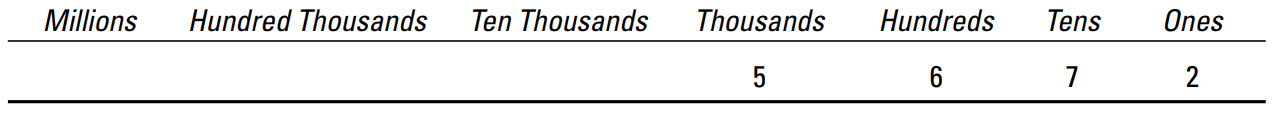# Getting in Place with Numbers and Digits

The number system used most commonly throughout the world is the Hindu-Arabic number system. This system contains ten digits (also called numerals), which are symbols like the letters A through Z. I’m sure you’re quite familiar with them: 1 2 3 4 5 6 7 8 9 0 Like letters of the alphabet, individual digits aren’t very useful. When used in combination, however, these ten symbols can build numbers as large as you like using place value. Place value assigns each digit a greater or lesser value depending upon where it appears in a number. Each place in a number is ten times greater than the place to its immediate right. Although the digit 0 adds no value to a number, it can act as a placeholder. When a 0 appears to the right of at least one non-zero digit, it’s a placeholder. Placeholders are important for giving digits their proper place value. In contrast, when a 0 isn’t to the right of any nonzero digit, it’s a leading zero. Leading zeros are unnecessary and can be removed from a number. Example: 1) Question: In the number 284, identify the ones digit, the tens digit, and the hundreds digit. 1) Answer: The ones digit is 4, the tens digit is 8, and the hundreds digit is 2. 2) Question: Place the number 5,672 in a table that shows the value of each digit. Then use this table and an addition problem to show how this number breaks down digit by digit. 2) Answer:The numeral 5 is in the thousands place, 6 is in the hundreds place, 7 is in the tens place, and 2 is in the ones place, so here’s how the number breaks down: 5,000 + 600 + 70 + 2 = 5,672Математичні настільні ігри